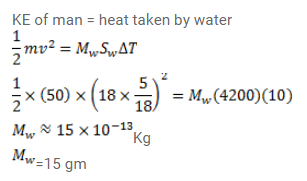# A 50 kg man is running at a speed of 18 km/h.Question:

A $50 \mathrm{~kg}$ man is running at a speed of $18 \mathrm{~km} / \mathrm{h}$. If all the kinetic energy of the man can be used to increase the temperature of water from $20^{\circ} \mathrm{C}$ to $30^{\circ} \mathrm{C}$, how much water can be heated with this energy?

Solution: